B Trees in Data Structures
Ratings:
(4)
Views:452## B Trees

### m-way search trees

#### Definition

An m-way search tree may be empty if it is not empty it is a tree that satisfies

### The following properties

In the corresponding extended search Tree, each inter-node has up to in children and between 1 and m-1 elements (External nodes contain no elements and have no children) Every node with p elements has exactly p+1 children Consider any node with p elements. Let k1----kp be the keys of these elements. The elements are ordered so that k1<k2 - -- -- kp. Let c0,C1…..C be the p+1 children of the node. The elements in the sub tree with root cp have keys larger than kp and those in the sub tree with root C; have keys larger than ki but smaller than ki+1,1<=i<p   Ex : A seven way search Tree G -> External nodes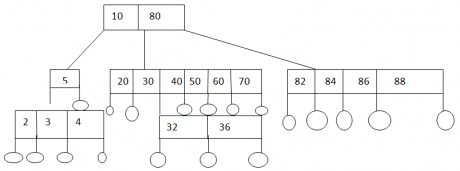### B trees of order m

Definition A B-tree in Data Structures at sizzler in is an m-way search tree if the B-tree is not empty, The corresponding extended tree satisfies the following properties The root has at least two children Fill internal nodes other than the root have at least [m/2] children. All external nodes are at the same level.

### Example of B-tree in Data Structures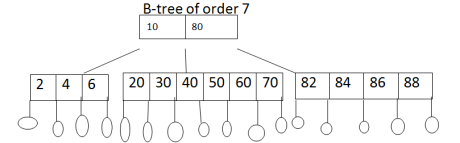Here m =7 m/2 =7/2 =3.5 =4 figure 2

### Insertion into a B tree

To insert an element into a B-tree we first search for the presence of an element with the same key. If such an element is found, The insert fails because duplicates are not permitted. When the search is unsuccessful, we attempt to insert the new element into the last internal node encountered on the search path. Ex: Insert 3into above figure(figure2) When inserting an element with key 3 into the B-tree of figure,we examine the root and its left child. We fall of the tree at the second external node of the left child, since the left child currently has three elements and can hold up to six, the new element any inserted into this node, the result is the B-tree of figure (3).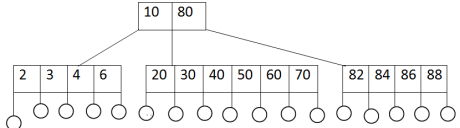E.g.:2 Insert 25 into figure3: This element is to go into the node [20, 30,40, 50, 60,70].However, this node is full when the new element needs to go into a full node, the full node is split.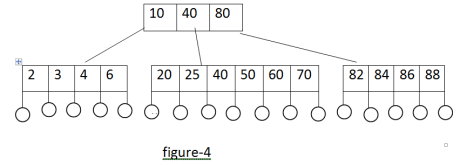### Deletion from a B tree

Deletion is first divided into two cases:

1. The element to be deleted is in a node whose children are external nodes (i.e. the element is in a leaf).
2. The element is to be deleted from a non-leaf case2 is transformed into case(1) by replacing the deleted element with either the largest element in its left neighboring sub tree or the smallest element in its right –neighboring sub tree. The replacing element is guaranteed to be in a leaf.

e.g. Delete 50 from the B tree of figure3   To delete 50 from the  B tree of figure 4, we write out the modified node [20, 30, 40, 60, 70 ]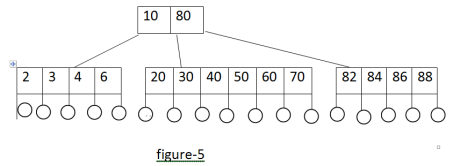E.g.2: Deleting the element with key 80 from the B tree of figure (3) Since the element is not in a leaf, we find a suitable replacement .The possibilities are the element with key 70 (i.e. the largest element in the left –neighboring sub tree) and 82 (i.e. the smallest element in the right- neighboring sub tree).When we use the 70, the problem of deleting this elements.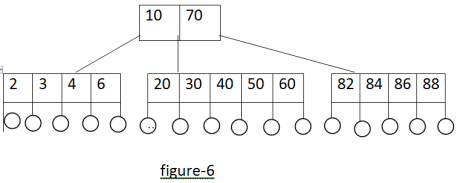E.g.3: Deleting element 25 from the B-tree of figure4 When the element is being deleted from a non-root node that has exactly the minimum number of elements, we try to replace the deleted element with an element from its nearest left sibling or right sibling. Notice that every node more other than the root has either a nearest left sibling or a nearest right sibling or both.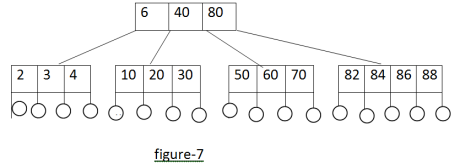E.g.: Deleting element 25 from B tree of figure 4. In our example the siblings [20, 30] and [50, 60, 70] and the element with the key 40 are merged into the single node [20, 30, 40, 50, 60, 70].The resulting B-tree is that of figure (8).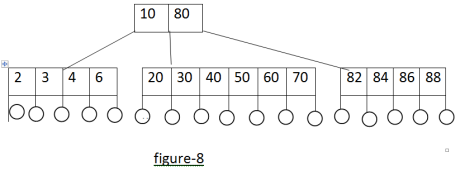Example Construct a B tree of order 3 using the following elements: 78, 52, 81, 40, 33, 90, 85, 20, 38 Solution## Deques

• A queue is a linear list in which elements can be added or removed at either end but not in the middle.
• The term deque is a contraction of the name double-ended queue.
• Such a structure can be represented by the following figure.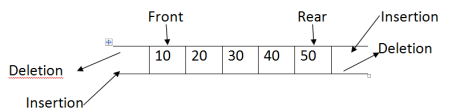• There are two variable of deque, namely, the input-restricted deque and output restricted deque.
• An input- restricted deque is a deque, which allows insertions at only one end of the list but allows deletion at both ends of the list.
• An output –restricted deque is a deque that allows deletions at only one end of the list but allows insertion at both ends of the list.
• A deque is a double –ended queue. You can insert items at either end and delete then from either end.
• If you restrict yourself to addqat beg() and delqatbeg(), then the deque acts like a stack .If you restrict yourself to addqatbeg() and delqatend(),then is acts like a queue.

### Program: Implementation of deque using arrays

#include<stdio.h> #include<conio.h> static int deque [max]; int front=-1; int rear=-1; // insert an element from void addqatbeg(int item) { int i; if(front ==0 &&rear == max-1) { printf(“\n Deque is full \n”); return; } if( front ==-1) { front =rear=0; Deque [front=item; return; } if (rear!=max-1) { int c= count(); int k=rear+1; for(i=1;i<=c; i++) { Deque[k]=Deque[k-1]; k- -; } Deque[k]=item; front=k; rear++; } else { front- -; Deque[front]=item; } } void addqatend(int item) { inti; if(front ==0 &&rear ==MAX-1) { printf(“\n Deque is full \n”); return; } if(front==-1) { rear=front=0; Deque[rear]=item; return; } if (rear==MAX-1) { int k=front-1; for(i=front-1;i<rear;i++) { k=i; if(k=MAX-1) Deque[k]=0; else Deque[k]=Deque[i+1]; rear - -; front- -; } rear++; Deque[rear]=item; }

#### //removes an element from the front end of deque

int delzatbeg() int item; if(front==-1) { printf(“\n Deque is empty \n”); return 0; } item =Deque[front]; Deque[front]=0; if (front==rear) front=rear=-1; else front++; return item; }

#### //removes an element from the rear end of the deque

int delqatend() { int item; if(front==-1) { printf(“\n Deque is empty\n”); return 0; } item=Deque[rear]; Deque[rear]=0; rear- -i; if(rear==-1) front=-1; return item; }

#### //display elements of a deque

void display() { int i; printf(“\n front à”); for(i=0;i<MAX; i++) printf(“%d”,Deque[i]); prinft(“ß rear”); }

#### //count the total number of elements in deque

int count() { int  c=0; int i; for(i=0;i<MAX;i++) { if(Deque[i]!=0)c++; } return c; } void main() { int n,i; addqatend(17); addqatbeg(10); addqatend(8); addqatbeg(-9); addqatend(13); addqatbeg(28); addqatend(14); addqatbeg(5); addqatend(25); addqatbeg(6); addqatend(21); addqatbeg(11); printf(“\n Elements in a deque:”); display(); n=count(); printf(“\n Total number of elements in deque :%d”,n); i=delqatbeg(); printf(“\n item extracted:%d”,i); i=delqatbeg(); printf(“\n item extracted:%d”,i); i=delqatbeg(); printf (“\ item extracted :%d”,i); i=delqatbeg(); printf(“\n item extracted:%d”,i); printf(“\n Elements in a Deque after addition:”); display(); i=delqatend(); printf(“\n item extracted :%d, i); i=delqatend(); printf(“/n item extracted:%d”,i); printf(“\n Elemens ina deque after deletion :”); display(); n=count(); printf(“\n Total number of elements in deque:%d”,n); } Output Elements is deque: front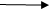6 5 29-9 10 17 8 13 14 25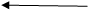rear Total number of elements in deque :10 item extracted :6 item extracted :5 item extracted:28 item extracted :-9     Elements in adeque after deletion: front0 0 0 0 10 17 13 14 25rear Elements in a deque after addition : fronto o 10 17 8 13 14 2516 7rear item extracted :7 item extracted :16 Elements in a deque after deletion: front0 0 10 17 8 13 14 25 0 0rear Total number of elements in deque: 6